# Right Triangle

Go back to  'Triangles'

 1 Introduction to a Triangle 2 What is Right Triangle? 3 Types of Right Triangles 4 Right Triangle Formula 5 Right Triangle Area 6 Tips and Tricks 7 Solved Examples on Right Triangle 8 Practice Questions on Right Triangle 9 Important Notes on Right Triangles 10 Maths Olympiad Sample Papers 11 Frequently Asked Questions (FAQs)

We at Cuemath believe that Math is a life skill. Our Math Experts focus on the “Why” behind the “What.” Students can explore from a huge range of interactive worksheets, visuals, simulations, practice tests, and more to understand a concept in depth.

Book a FREE trial class today! and experience Cuemath’s LIVE Online Class with your child.

## Introduction to a Triangle

Triangles are three-sided closed figures.

Drag the vertices in the simulation below to see the types of triangles and its elements.

Explore all types of triangles

## What is a Right Triangle?

The definition for a right triangle states that if one of the angles of a triangle is a right angle ($$90^\circ$$), the triangle is called a right-angled triangle or simply, a right triangle.

Triangle ABC is a right triangle, where: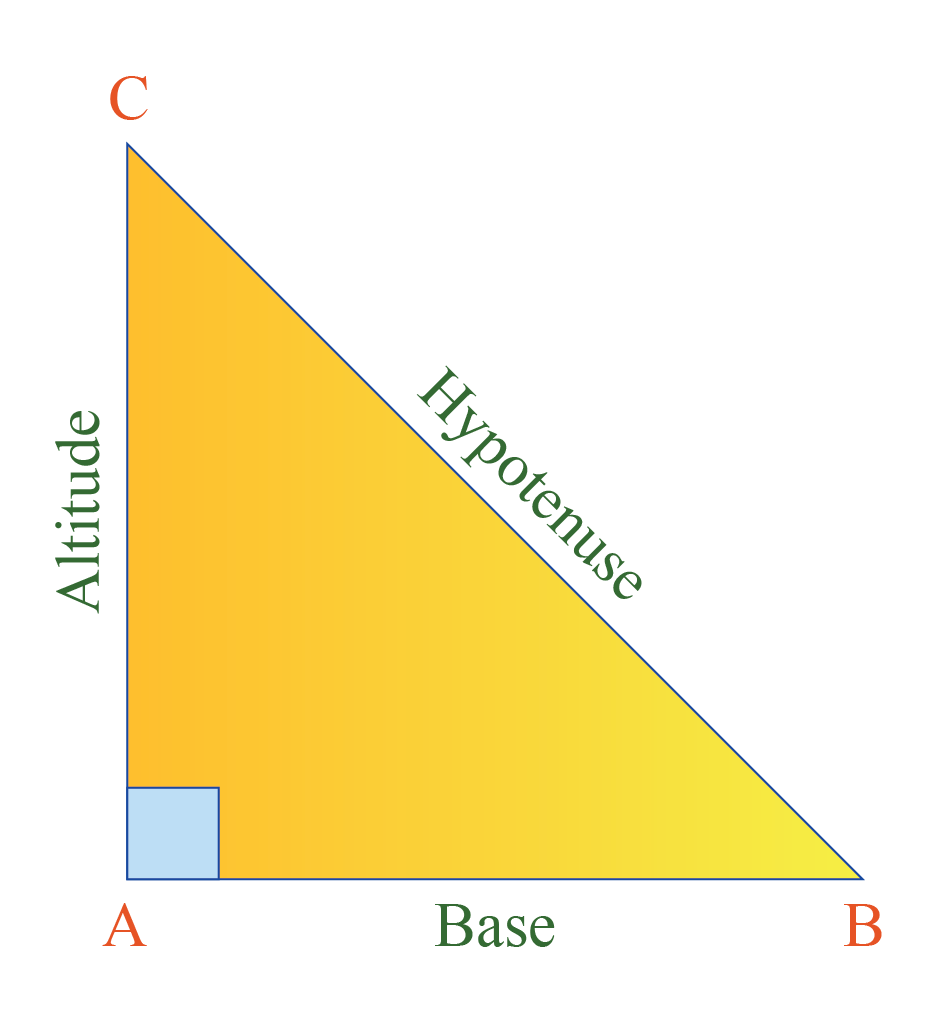• AC is the height, altitude or perpendicular
• AB is the base
• $$\text{AC} \perp \text{AB}$$
• $$\angle A=90^\circ$$
• the side BC opposite to the right angle is called the hypoteneuse and it is the longest side of the right triangle.

Now, let's explore a right triangle visually.

Drag the vertices A and C in the simulation below

Observe the right triangle angles.

## Types of Right Triangles

We learnt that one of the angles in a right triangle is $$90^\circ$$

This implies that the other two angles in the triangle will be acute angles.

There are a few special right triangles namely isosceles right triangle and scalene right triangle.

### Isosceles Right Triangle

An isosceles right triangle is called as a 90$$^\circ$$-45$$^\circ$$- 45$$^\circ$$ triangle.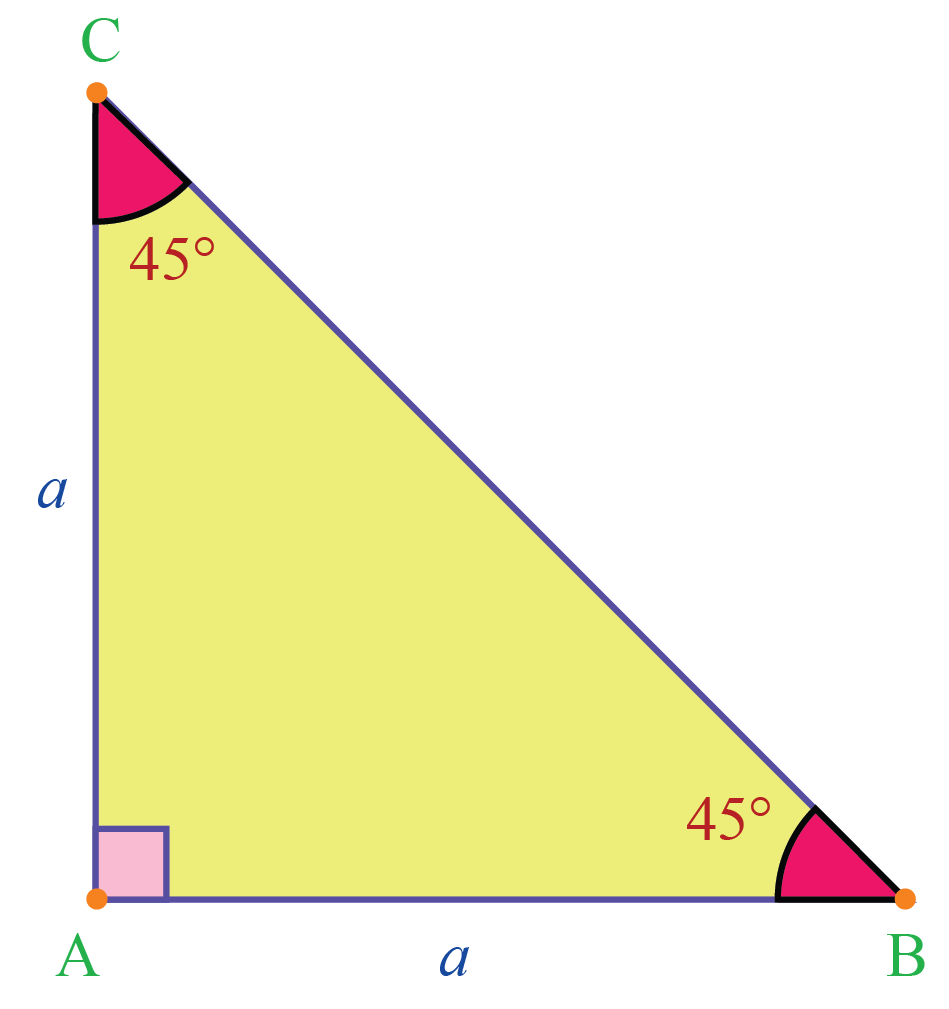In triangle ABC,

• $$\angle A = 90^\circ$$; by right triangle definition, triangle ABC is a right triangle.
• AB = AC; since two sides are equal, the triangle is also an isosceles triangle.

Since AB = AC, the base angles are equal.

We know that the sum of the angles of a triangle is 180$$^\circ$$

Hence, the base angles add up to 90$$^\circ$$ which implies that they are 45$$^\circ$$ each.

So in an isosceles right triangle, angles will always be 90$$^\circ$$-45$$^\circ$$- 45$$^\circ$$

### Scalene Right Triangle

A scalene right triangle is a triangle where one angle is 90$$^\circ$$ and other two angles add up to 90$$^\circ$$.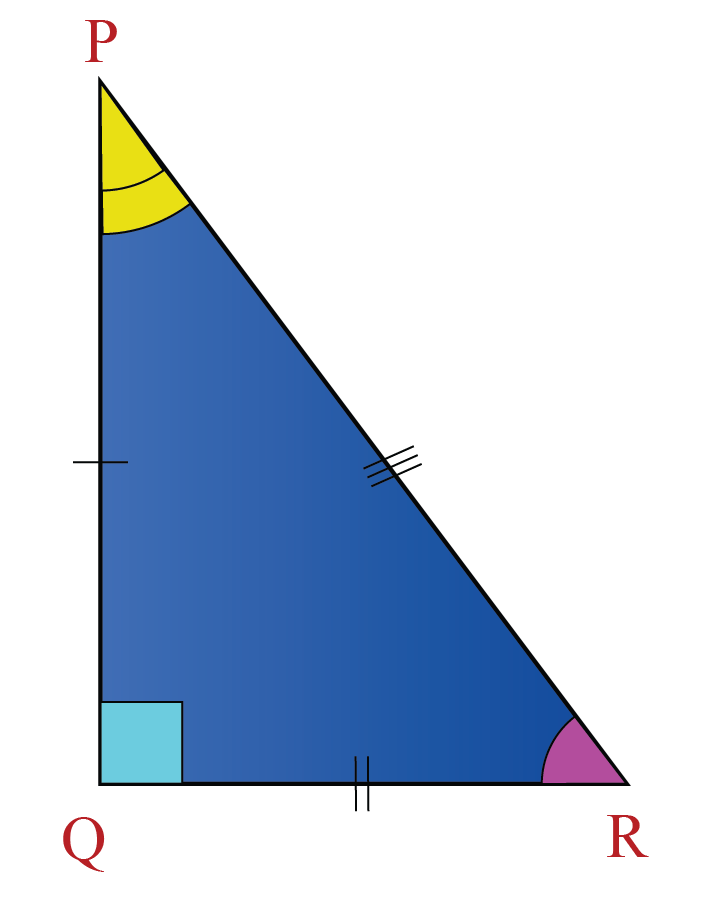In the triangle PQR,

• $$\angle Q =90^\circ$$, hence, it is a right triangle.
• $$\text{PQ} \neq \text{PR} \neq \text{QR}$$, hence, it is a scalene triangle.

There is also a special $$30^\circ$$-$$60^\circ$$-$$90^\circ$$ right triangle where the ratio of the triangle's longest side to its shortest side is $$2:1$$

The side opposite to the $$30 ^\circ$$ angle is the shortest side.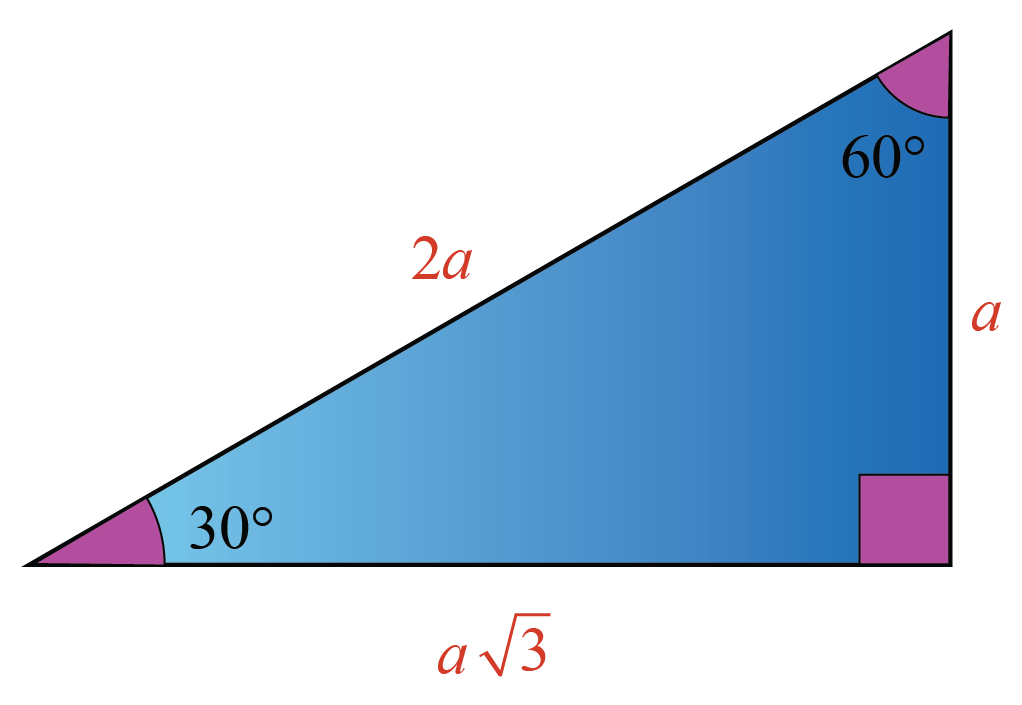## Right Triangle Formula

The great Greek philosopher, Pythagoras, derived an important formula for a right triangle.

The formula states that in a right triangle, the square of the hypoteneuse is equal to the sum of the squares of the other two legs.

It was named after him as Pythagoras theorem.

The right triangle formula can be represented in the following way.

In a right triangle,

 \begin{align} \text{Hypotenuse}^2 &=\text{Base}^2 +\text{Altitude}^2\end{align}

Let's explore this formula with the help of a simulation.

Click 'Go' to see the Geometric proof of the Pythagoras Theorem.

### Pythagorean Triplet

The three numbers which satisfy the above equation is known as the Pythagorean triplet.

For example, (3, 4, 5) is a Pythagorean triplet because we know that $$3^2 = 9$$, $$4^2 = 16$$  and $$5^2 = 25$$

We know that $$9 +16 = 25$$

Therefore, $$3^2 + 4 ^2 = 5^2$$

These three numbers satisfying this condition are called the Pythagorean triplet.

### Perimeter of a Right Triangle

The perimeter of a right triangle is the sum of the measures of all the 3 sides.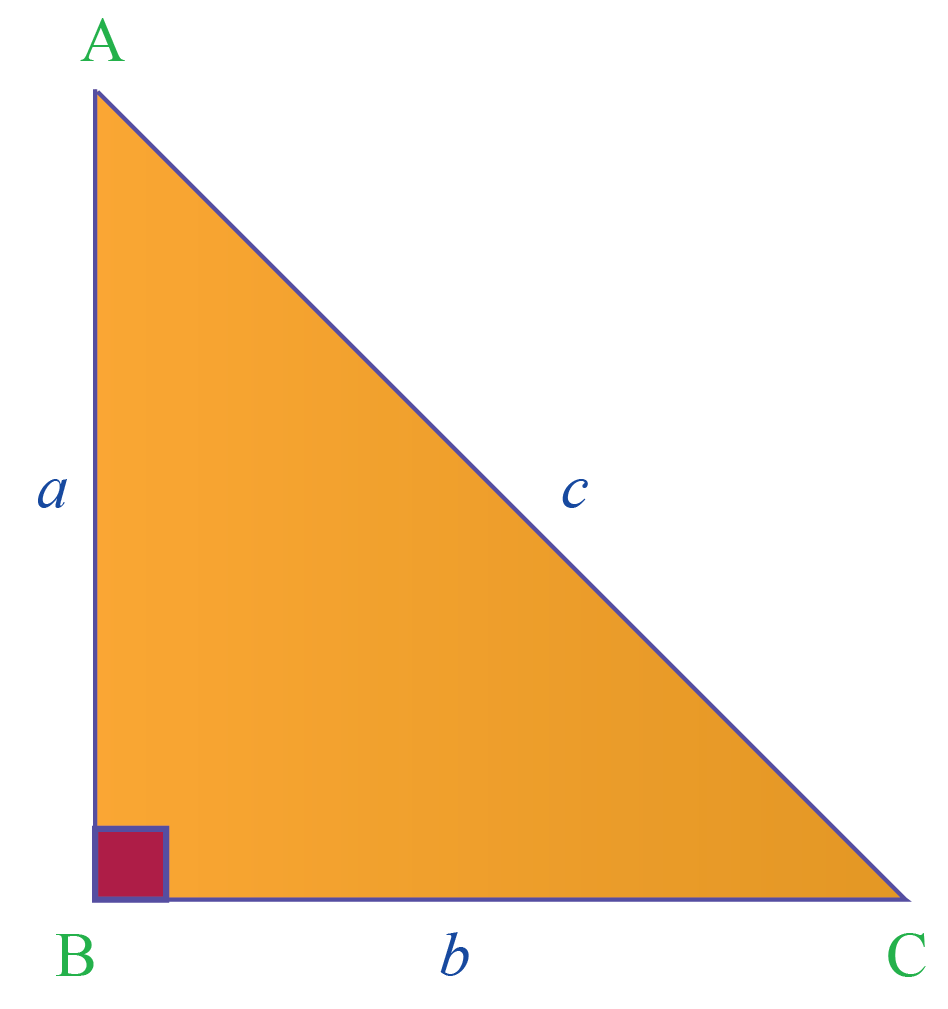In the given right triangle ABC, the sides are of length $$a$$,$$b$$, and $$c$$ units.

The formula for the perimeter of a right triangle is given by:

 Perimeter of a right triangle = $$a +b + c$$

## Right Triangle Area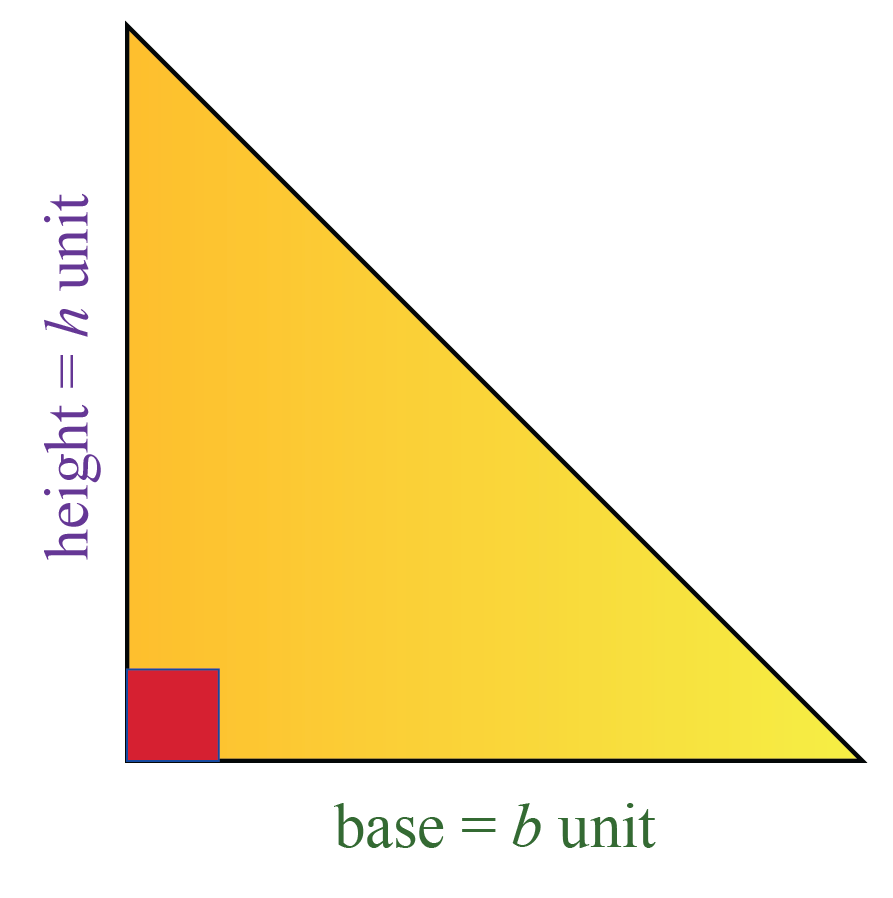Applying the right triangle definition, the area of a right triangle is given by the formula:

 \begin{align}\frac{1}{2} \times \text{base} \times \text{height}\end{align}

Use the following right triangle calculator to calculate the area.

Enter the value of the base and height in the space provided.Tips and Tricks
1. The measures of a right triangle will always satisfy the Pythagorean theorem.
2. In a right triangle, the hypoteneuse is the side opposite to right angle and it is the longest side of the triangle.
3. The other two legs are perpendicular to each other; one is the base and the other is the height.

Help your child score higher with Cuemath’s proprietary FREE Diagnostic Test. Get access to detailed reports, customised learning plans and a FREE counselling session. Attempt the test now.

## Solved Examples

 Example 1

Can a right triangle have 11 cm, 60 cm and 61 cm as its dimensions?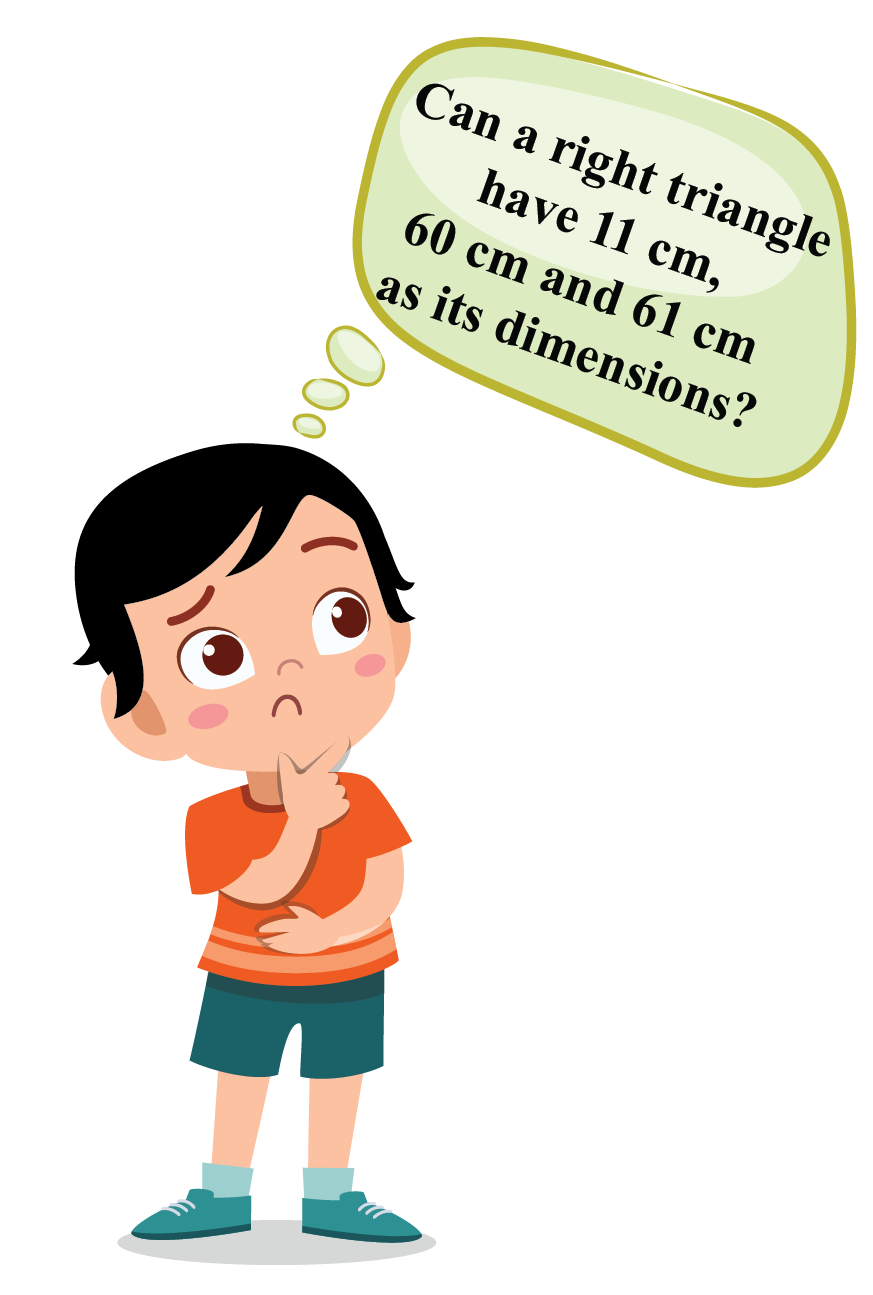Solution:

If 11, 60 and 61 are a Pythagorean triplet, they will form a right triangle.

$$11^2 = 121$$; $$60^2 = 3600$$; $$61^2= 3721$$

We can see that:

$$121 +3600 = 3721$$

Hence, the given numbers are a Pythagorean triplet and can be the dimensions of a right triangle.

 $$\therefore$$11 cm, 60 cm and 61 cm forms a right triangle.
 Example 2

Find the area of a right triangle whose base is 12 cm and height is 5 cm.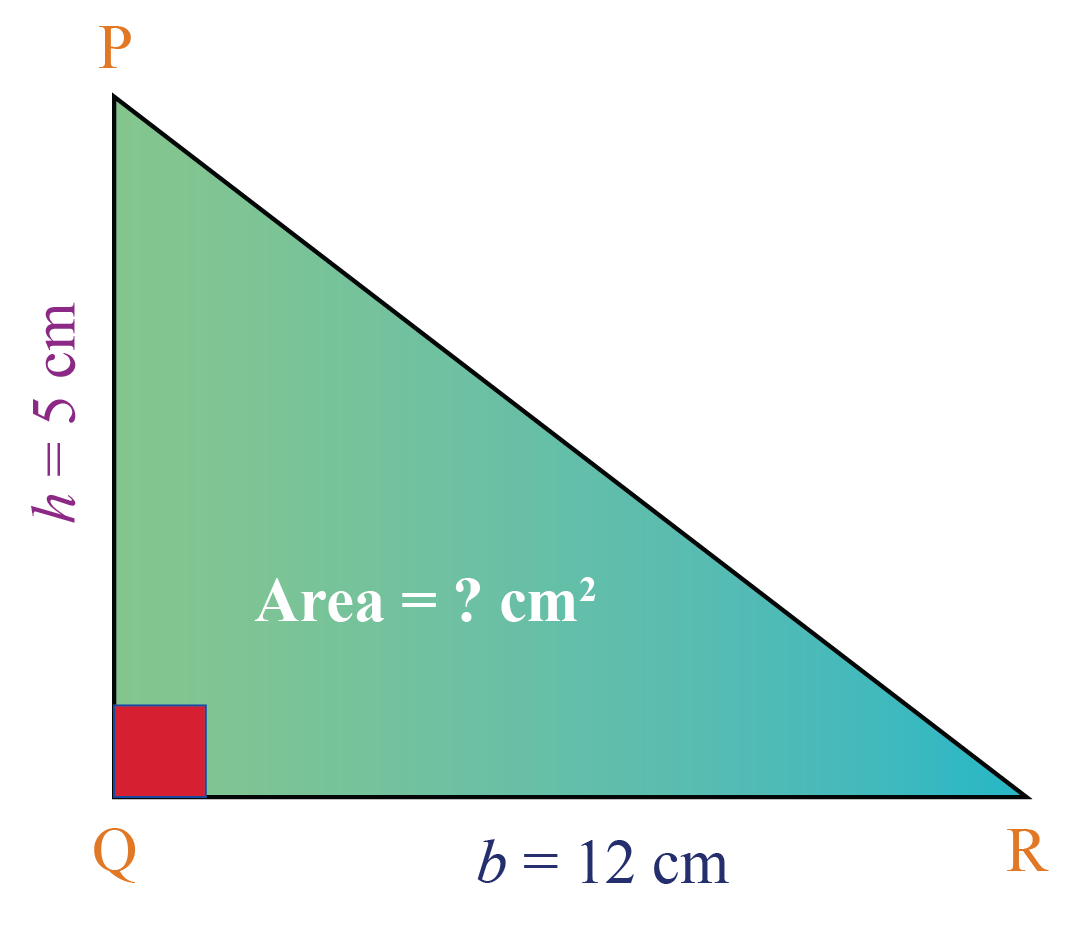Solution:

Area of a triangle is given by the formula:

\begin{align}\frac{1}{2} \times b \times h\end{align}

Substituting b = 12 cm and h = 5 cm, we have

\begin{align} \text{Area of the triangle} &=\frac{1}{2} \times 12 \times 5\ \\ &= 30 \text{ cm}^2 \\ \end{align}

 $$\therefore$$ Area of the triangle = $$30 \text{ cm}^2$$
 Example 3

The hypoteneuse of a right isosceles triangle is 8 units. Find its area.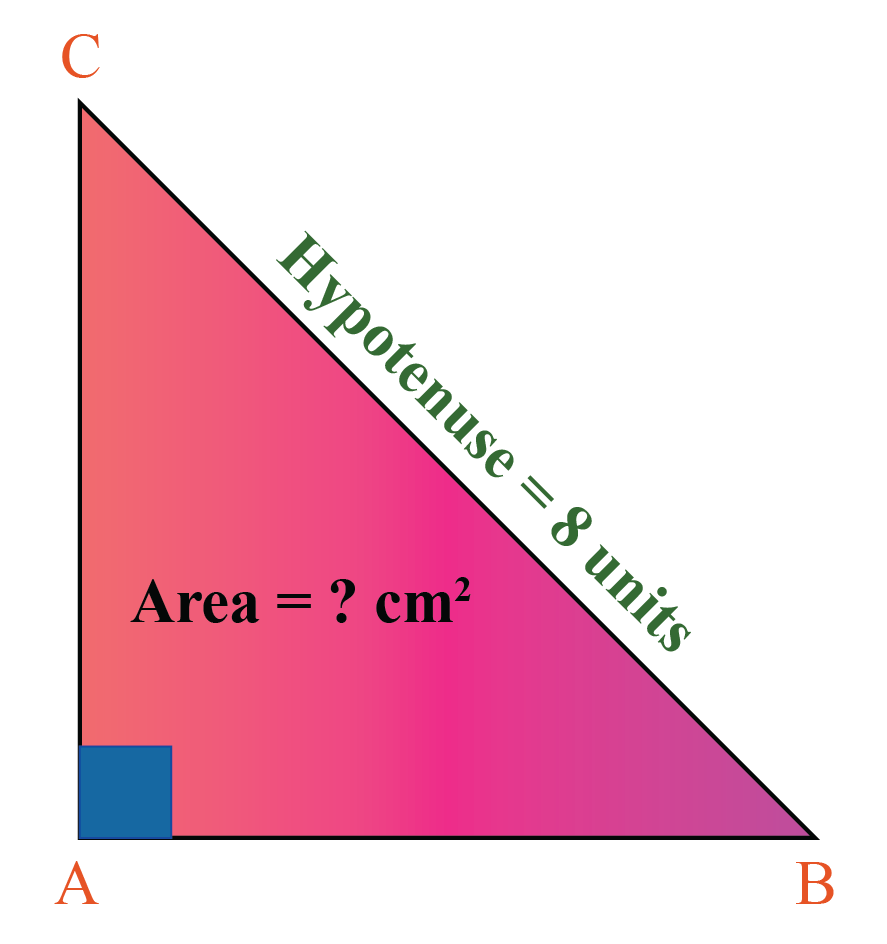Solution:

In a right isosceles triangle, we know that:

$$\text{Hypotenuse}^2$$ = $$\text{Base}^2$$ + $$\text{Altitude}^2$$

Since the triangle is isoscles, the height and base measures the same.

Let us keep it as $$x$$.

Therefore,

\begin{align} 8^2&= x^2 +x^2 \\ 64 &= 2x^2 \\ \Rightarrow x^2&= 32 \\ x &= 5.65 \end{align}

We now have base = 5.65 cm and height = 5.65 cm.

Area of the triangle:

\begin{align}\frac{1}{2} \times b \times h\end{align}

Let's substitute the value of base and height in the formula.

Area of the triangle:

\begin{align} \ &=\frac{1}{2} \times 5.65 \times 5.65\ \\ &= 15.96 \text{ cm}^2 \\ \end{align}

 $$\therefore$$ Area of this triangle is 15.96 cm2
 Example 4

The perimeter of a right triangular swimming pool is 720 m. The three sides of the pool are in the ratio 3:4:5

Find the area of the pool.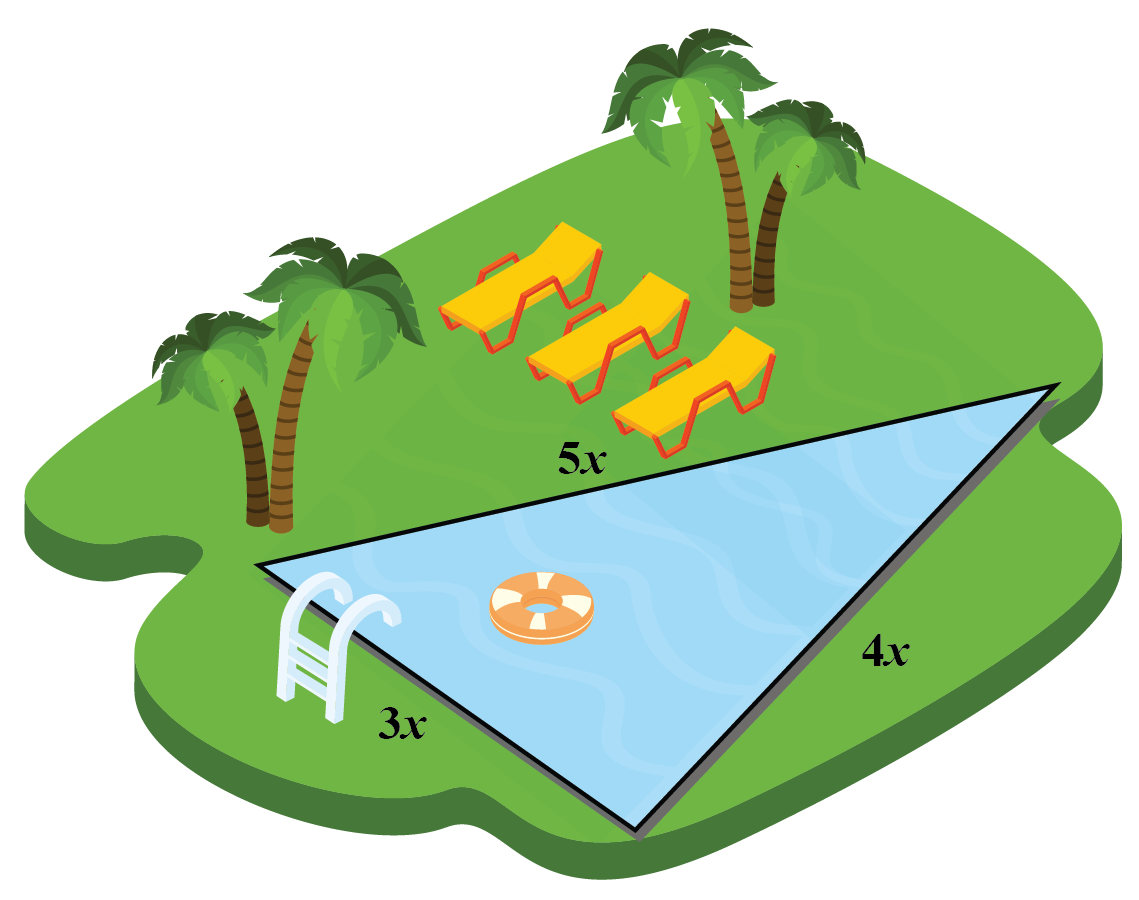Solution:

Perimeter of the triangle is the sum of the measures of all the sides.

Therefore,

\begin{align} 3x +4x+5x &= 720\\ 12x &= 720\\ x &= 60 \\ \end{align}

The sides of the triangle are:

180 cm, 240 cm and 300 cm

Since,

$$180^2 + 240^2 = 300^2$$

The sides form a right triangle with hypoteneuse 400 cm.

Therefore, the area of the swimming pool is:

\begin{align} \ &=\frac{1}{2} \times 180 \times 240\ \\ &= 21600 \text{ m}^2 \\ \end{align}

 $$\therefore$$ Area of the swimming pool = 21600 m2
 Example 5

Find the value of a and b in the given figure.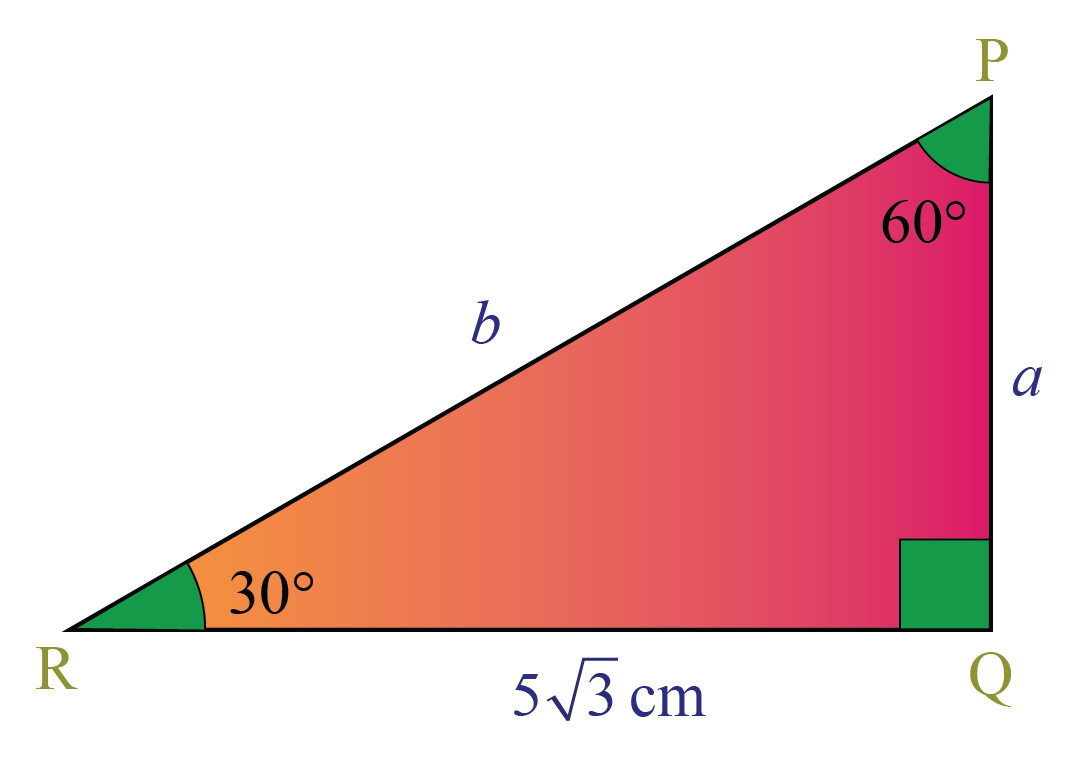Solution:

The given triangle ($$30^\circ$$-$$60^\circ$$-$$90^\circ$$) is a special right triangle where the hypoteneuse is twice the shortest side.

The side opposite to $$30^\circ$$ is the shortest side and the longest side is twice the shortest side.

Therefore,

$$b = 2a$$

The other side is $$a\sqrt{3}$$ which is equal to 5$$\sqrt{3}$$ cm as given in the diagram.

Therefore,

a = 5 cm

This implies:

b =  10 cm

 $$\therefore$$ a = 5 cm and b = 10 cm

CLUEless in Math? Check out how CUEMATH Teachers will explain Right triangle to your kid using interactive simulations & worksheets so they never have to memorise anything in Math again!

Explore Cuemath Live, Interactive & Personalised Online Classes to make your kid a Math Expert. Book a FREE trial class today!

## Practice Questions

Here are a few activities for you to practice.

IMO (International Maths Olympiad) is a competitive exam in Mathematics conducted annually for school students. It encourages children to develop their math solving skills from a competition perspective.

## 1. What is a right triangle?

A triangle in which one of the angle measures is 90$$^\circ$$ is called a right-angled triangle or right triangle.

## 2. What are the different types of right triangles?

An isosceles right triangle is a right triangle where the angles of the triangle are 90$$^\circ$$, 45 $$^\circ$$ and 45$$^\circ$$

A scalene right triangle is a right triangle where one angle is 90$$^\circ$$ and the other two angles add up to 180$$^\circ$$

30$$^\circ$$-60$$^\circ$$-90$$^\circ$$ triangle is another interesting right triangle where the ratio of the triangle's longest side to its shortest side is 2:1

## 3. What is the measure of the angles in a right triangle?

A right triangle has one of its angles as 90$$^\circ$$

The other two angles of the triangle add up to 180$$^\circ$$

More Important Topics
Numbers
Algebra
Geometry
Measurement
Money
Data
Trigonometry
Calculus